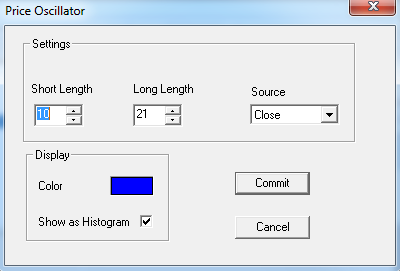# Price Oscillator

User can add Price Oscillator indicator to their chart by right click the chart and select Study Config to open the study configure window first, then select the “Price Oscillator” from the study list and add it into the Studies In Chart field.Price Oscillator Configure window allows user to modify the Price Oscillator chart, such as change the study period/length and line’s color. User can open this Price Oscillator Configure window either by click the “Config” button or double click the study “Price Oscillator” under Studies In Chart section.

## Explanation of Each Field

·         Settings section allows user to modify the study parameters for Price Oscillator study.

Ø  Short Length: Enter the short length for Price Oscillator study.

Ø  Long Length: Enter the long length for Price Oscillator study.

Ø  Source: Use the dropdown menu to select the study source for the Price Oscillator study.

§  Open: Use the open price data to calculate the Price Oscillator.

§  High: Use the highest price data to calculate the Price Oscillator.

§  Low: Use the lowest price data to calculate the Price Oscillator.

§  Close: Use the close price data to calculate the Price Oscillator.

§  (H+L)/2: Use this equation to calculate the price first, then use those price data to calculate the Price Oscillator.

§  (H+L+C)/3: Use this equation to calculate the price first, then use those price data to calculate the Price Oscillator.

§  (O+H+L+C)/4: Use this equation to calculate the price first, then use those price data to calculate the Price Oscillator.

§  (H+L+C+C)/4: Use this equation to calculate the price first, then use those price data to calculate the Price Oscillator.

(Hint, O means Open Price, H means Highest Price, L means Lowest Price, and C means Close Price. And in (H+L+C+C)/4, both Cs are refer to Close Price, so same as (H+L+2C)/4.)

·         Display Section allows client to modify the chart style and its colors.

Ø  Color: Click on the color field allows user to change the study color.

Ø  Show as Histogram: Check off this box to show it as a histogram.